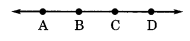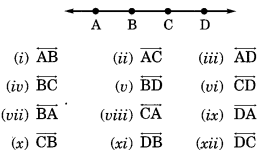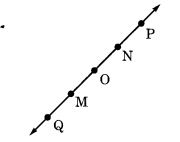# NCERT Solutions for Class 6 Maths Chapter 4 Basic Geometrical Ideas

ncert textbook

## NCERT Solutions for Class 6 Maths Chapter 4 Basic Geometrical Ideas

NCERT Solutions for Class 6 Maths Chapter 4 Basic Geometrical Ideas Ex 4.1

Exercise 4.1

Question 1.
Use the figure to name:
(a) Five points
(b) A line
(c) Four rays
(d) Five line segments
Solution:
(a) Five points are: O, B, C, E and D(b) Name of the line is$\overleftrightarrow { DB }$or$\overleftrightarrow { BD }$.
(c) Four rays are:$\overrightarrow { OC }$,$\overrightarrow { OB }$,$\overrightarrow { OE }$and$\overrightarrow { OD }$
(d) Five line segments are:$\overline { OE }$,$\overline { ED }$,$\overline { OD }$,$\overline { OB }$and$\overline { EB }$.

Question 2.
Name the line given in all possible (twelve) ways, choosing only two letters at a times from the four given.Solution:
The given lines can be named as follows:Question 3.
Use the figure to name:
(a) Line containing point E.
(b) Line passing through A.
(c) Line on which 0 lies.
(d) Two pairs of intersecting lines.Solution:
(a)$\overleftrightarrow { EF }$
(b)$\overleftrightarrow { AE }$
(c)$\overleftrightarrow { BC }$or$\overleftrightarrow { BO }$
(d)$\overleftrightarrow { CO }$or$\overleftrightarrow { AE }$or$\overleftrightarrow { AE }$or$\overleftrightarrow { EF }$

Question 4.
How many lines can pass through
(a) one given point?
(b) two given points?
Solution:
(a) Infinitely many lines can pass through a given points.
(b) Only one line can pass through two given points.

Question 5.
Draw a rough figure and label suitably in each of the following cases:
(a) Point P lies on$\overline { AB }$.
(b)$\overleftrightarrow { XY }$and$\overleftrightarrow { PQ }$intersect at M.
(c) Line L contains E and F but not D.
(d)$\overleftrightarrow { OP }$and$\overleftrightarrow { OQ }$meet at O.
Solution:Question 6.
Consider the following figure of line MN. Say whether following statements are true or false in context of the given figure.(a) Q, M, O, N, P are points on the line$\overleftrightarrow { MN }$.
(b) M, O, N are points on a line segment$\overline { MN }$.
(c) M and N are end points of line segment$\overline { MN }$.
(d) O and N are end points of line segment$\overline { OP }$.
(e) M is one of the end points of line segment$\overline { QO }$.
(f) M is point on ray$\overrightarrow { OP }$.
(g) Ray$\overleftrightarrow { OP }$is different from ray$\overrightarrow { QP }$.
(h) Ray$\overleftrightarrow { OP }$is same as ray$\overrightarrow { OM }$.
(i) Ray$\overrightarrow { OM }$is not opposite to ray$\overleftrightarrow { OP }$.
(j) O is not an initial point of$\overleftrightarrow { OP }$.
(k) N is the initial point of$\overrightarrow { NP }$and$\overrightarrow { NM }$.
Solution:
(a) True
(b) True
(c) True
(d) False
(e) False
(f) False
(g) True
(h) False
(i) False
(j) False
(k) True